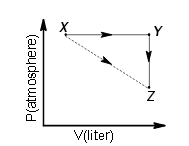×#### Thank you for registering.

One of our academic counsellors will contact you within 1 working day.

Click to Chat

1800-1023-196

+91-120-4616500

CART 0

• 0

MY CART (5)

Use Coupon: CART20 and get 20% off on all online Study Material

ITEM
DETAILS
MRP
DISCOUNT
FINAL PRICE
Total Price: Rs.

There are no items in this cart.
Continue Shopping• Complete JEE Main/Advanced Course and Test Series
• OFFERED PRICE: Rs. 15,900
• View Details

```Question:1) A piston filled with 0.04 mol of an ideal gas expands reversibly from 50.0 mL to 375 mL at a constant temperature of 37.00 C. As it does so, it absorbs 208J of heat. The values of q and w for the process will be:

(R = 8.314 J/mol K) (ln 7.5 = 2.01) (IIT-JEE 2013)

(1) q = – 208 J, w = – 208 J

(2) q = – 208 J, w = + 208 J

(3) q = + 208 J, w = + 208 J

(4) q = + 208 J, w =– 208 J

Solution:

For isothermal process, ? U = 0

Process is isothermal reversible expansion, hence dE =0

U = 0.

So, Using 1st law of thermodynamics

q = -W

or

w = -q = -208 JQuestion : 2) For an ideal gas, consider only P-Vwork in going from an initial state X to the final state Z. The final state Z can be reached by either of the two paths shown in the figure. Which of the following choice(s) is(are) correct?

[Take ?S as change in entropy and w as work done]  (IIT-JEE 2012)1) ΔS x→z = ΔS x→y + ΔS y→z

2) w x→z = w x→y + w y→z

3) w x→y→z = w x→y

4) ΔS x→y→z = ΔS x→y

A & C

Solution:

Entropy  being a state function shows additive property.

Hence,

ΔS x→z = ΔS x→y + ΔS y→z

For the process Y →Z pressure is constant.

W = -pΔV

At constant pressure

W =0

Hence, w x→y→z = w x→y

Question: 3 ) The standard enthalpies of formation of CO2(g), H2O(l) and glucose(s) at 25 0C are–400 kJ/mol, –300 kJ/mol and –1300 kJ/mol, respectively. The standard enthalpy of combustion per gram of glucose at 250C is  (IIT JEE 2013)

a. +2900kJ

b. -2900 kJ

c. +16.11 kJ

d. -16.11 kJ

Solution:

Formation of CO2:

C( graphite) + O2 (g)→ CO2 (g)    ΔH1= -400 kJ ………….(1)

Formation of H2O:

H2( g) + O2 (g) → H2O (l)    ΔH2= -300 kJ…………..(2)

Formation of C6H12O6:

6C( graphite) + 3O2 (g) + 6H2( g)  → C6H12O6 (s)    ΔH3= -1300 kJ ………(3)

6×Equation (1) + 6×Equation (2) - Equation (3)

We get,

C6H12O6 (s)    + 6O2 (g) → 6CO2 (g) + 6H2O (l)    ……….(4)

ΔH4= 6×(-400) + 6×(-300)- (-1300) = 2900 kJ/mol

Equation number 4 represents the equation for combustion of 1 mole of glucose so, the enthalpy change for the reaction i.e. ΔH4 is the enthalpy of combustion of glucose.

Enthalpy change for combustion of 1 mole i.e. 180 gm glucose = 2900 kJ

So, enthalpy change for combustion of 1 gm glucose =  2900/180 = 16.11 kJ.

Hence, the correct option is C.
```### Course Features

• 728 Video Lectures
• Revision Notes
• Previous Year Papers
• Mind Map
• Study Planner
• NCERT Solutions
• Discussion Forum
• Test paper with Video Solution